# Technical Guide

TOP > 晶体产品 > Part Numbering System > Technical Guide

## Types of crystal cut

### Relation among crystal cut, mode of vibration and frequency range

Crystal Cut Mode of vibration Frequency range (kHz) Capacitance ratio
(C0/C1)
AT Thickness shear Fundamental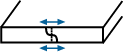800~5,000
2,000~80,000
450~300
220
3rd overtone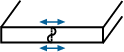20,000~90,000 n2 x 250
n: Overtone order
5rd overtone 40,000~150,000
7rd overtone 70,000~210,000
BT Thickness shear Fundamental Thickness shear Fundamental 3,000~30,000 650
XY Flexural (Tuning fork)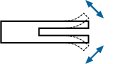16~150 425~800
Extensional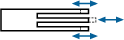600~3,000 400
DT Face shear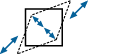100~500 400
CT 150~850 350
SL 180~700 400

## Crystal unit equivalent circuit

A crystal unit in the main resonance frequency may be expressed as an electrical equivalent circuit: a circuit ordinarily composed of a series circuit consisting of an inductance, capacitance and resistance, and a capacitance connected in parallel to the series circuit as shown in the drawing.

Here, C0, which is commonly known as the shunt capacitance, comprises an inter-electrode static capacitance to which the inter-terminal stray capacitance is added.

L1 and C1 are the equivalent constants of the crystal unit viewed as an electrical and mechanical oscillation system. Since both constants are determined by such factors as the type of cut, cutting angle, dimensions of the crystal blank, and construction of the electrodes, and are thus reproducible, crystal units can be manufactured with high precision.

R1, which denotes oscillation loss, is governed by conditions such as processing, storage, and dimensions of the crystal unit.

L1 is referred to as motional inductance, C1 is referred as motional capacitance, and R1 is referred as motional (series) resistance.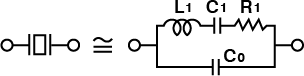L1 Motional inductance Motional capacitance Motional(series)resistance Shunt capacitance

The electrical equivalent circuit, composed of L1, C1, R1 and C0, all of which are correlated, may be expressed by the following equation.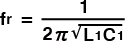Shown below are some equations by which the performances of crystal units are expressed.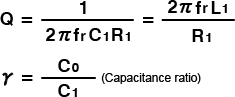## Frequency-temperature characteristics

### Correlation between Crystal cutting angle and Frequency-temperature characteristics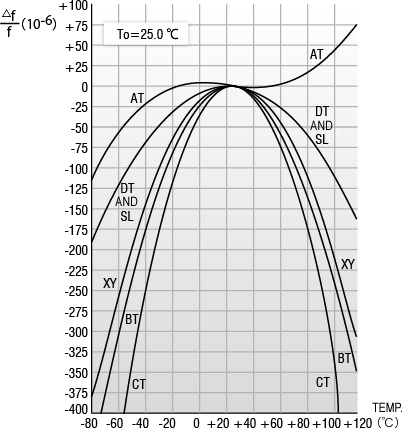### Frequency-temperature characteristics of Tuning Fork Crystal Unit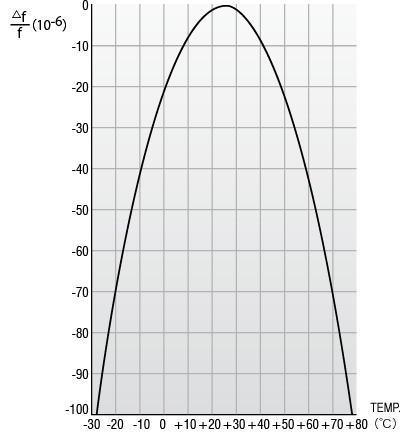### Correlation between Crystal cutting angle and Frequency-temperature characteristics of AT-cut Crystal Unit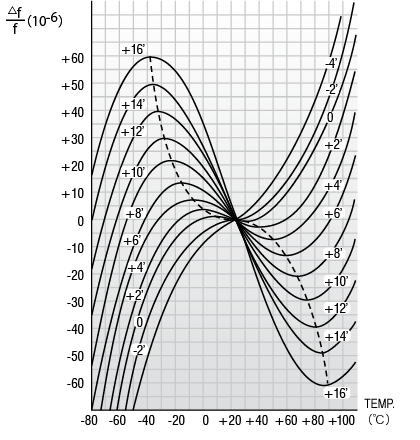## Test Circuit

### CSX-750F/SSX-750P SERIES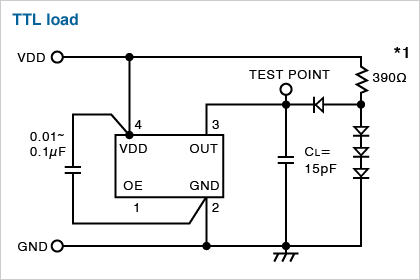*1

390Ω CSX-750(FC) SSX-750(PT, PK)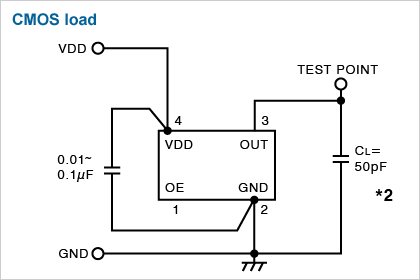*2

50pF CSX-750(FC) CSX-750(FB, FJ) SSX-750(PC, PD) SSX-750(PB, PJ), CSX-252F

### Current Consumption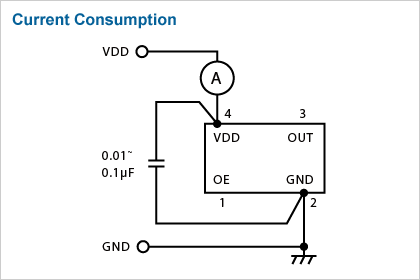## Output wave-form

### Oscillators output wave-form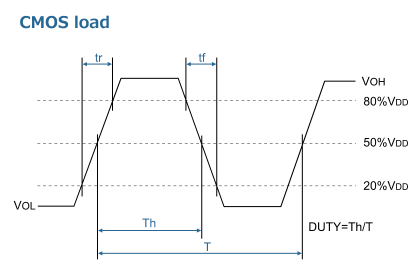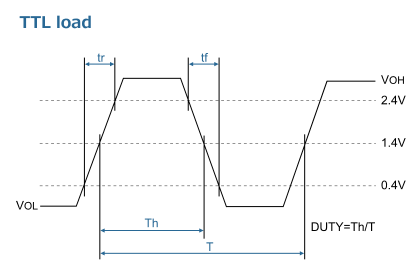### Measurement conditions

• Oscilloscope・Input impedance: No less than 1 MΩ・Input capacitance: No more than 15 pF・Band width: No less than 500 MHz・Make the grounding lead of the probe as short as possible.
• The CL includes the probe capacitance.
• Grounding should be single-point grounding.
• Supply voltage impedance should be as low as possible. Rise time from 0V to 0.9Vdd is to be more than 150 μs.
• Use an ammeter with small internal impedance.